# Иностранная литература и современность: General Statistics: Ch 4 Quiz Flashcards

Set Details Share
created 9 years ago by GreenHero64
136,786 views
This course is designed to acquaint the student with the principles of descriptive and inferential statistics. Topics will include: types of data, frequency distributions and histograms, measures of central tendency, measures of variation, probability, probability distributions including binomial, normal probability and student's t distributions, standard scores, confidence intervals, hypothesis testing, correlation, and linear regression analysis. This course is open to any student interested in general statistics and it will include applications pertaining to students majoring in athletic training, pre-nursing and business.
updated 9 years ago by GreenHero64
College: First year, College: Second year, College: Third year, College: Fourth year
Subjects:
statistics, mathematics, probability & statistics
Page to share:
Embed this setcancel
COPY
code changes based on your size selection
Size:
X

1

In a certain weather forecast comma the chances of a thunderstorm are stated as ​"1 in 16."

Express the indicated degree of likelihood as a probability value between 0 and 1 inclusive.

The probability is 0.063.

1/16 = 0.0625

2

For a certain horse race, the odds in favor of a certain horse finishing in second place are given as 51 to 49.

Express the indicated degree of likelihood as a probability value between 0 and 1 inclusive.

The probability is 0.51.

51 ÷ (51 + 49)

= 51/100 = 0.51

3

Assume that 1200 births are randomly selected and exactly 607 of the births are girls.

Use subjective judgment to determine whether the given outcome is​ unlikely.

Determine whether it is unusual in the sense that the result is far from what is typically expected.

It is unlikely because the probability of this particular outcome is very​ small, considering all of the other possible outcomes.

Recall that an event is unlikely if its probability is very​ small, such as 0.05 or less. Consider the complement of the given outcome and how likely it is.

It is not unusual because 607 is about the number of girls expected.

4

Assume that 900 births are randomly selected and exactly 219 of the births are girls.

Use subjective judgment to determine whether the given outcome is​ unlikely.

Determine whether it is unusual in the sense that the result is far from what is typically expected.

It is unlikely because there are many other possible outcomes that have similar or higher probabilities.

It is unusual because it is not about 450 as expected.

5

You are certain to get a black or a red card when selecting cards from a shuffled deck.

Express the indicated degree of likelihood as a probability value between 0 and 1 inclusive.

The probability is 1.

6

It is impossible to get 8 aces when selecting cards from a shuffled deck.

Express the indicated degree of likelihood as a probability value between 0 and 1 inclusive.

The probability is 0.

7

You toss a coin and randomly select a number from 0 to 9.

What is the probability of getting tails and selecting a 5?

0.05

There are ten numbers, and two coin choices, therefore 20 possibilities.

1/20 = 0.05

8

A random number generator is used to select a number from 1 to 100. What is the probability of selecting the number 149?

0

9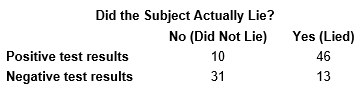Refer to the sample data for polygraph tests shown below.

If one of the test subjects is randomly selected, what is the probability that the subject is not lying?

Is the result close to the probability of 0.440 for a negative test result?

The probability that a randomly selected polygraph test subject was not lying is 0.410.

Yes, because there is less than a 0.050 absolute difference between the probability of a true response and the probability of a negative test result.

10

Among respondents asked which is their favorite seat on a plane, 487 chose the window seat, 10 chose the middle seat, and 310 chose the aisle seat.

What is the probability that a passenger prefers the middle seat?

Is it unlikely for a passenger to prefer the middle seat?

If so, why is the middle seat so unpopular?

The probability that a passenger prefers the middle seat is 0.012.

Yes, because the probability that a passenger prefers the middle seat is less than 0.05.

The middle seat lacks an outside view, easy access to the aisle, and a passenger in the middle seat has passengers on both sides instead of on one side only.

11

In a certain weather forecast, the chance of a thunderstorm is stated as 14%.

Express the indicated degree of likelihood as a probability value between 0 and 1 inclusive.

The probability is 0.14.

12

If A denotes some event, what does Ā denote?

If P(A) = 0.994, what is the value of P(Ā)?

If P(A) = 0.994, is Ā unusual?

Event Ā denotes the complement of event A, meaning that Ā consists of all outcomes in which event A does not occur.

P(Ā) = 0.006

Yes, Ā is unusual.

13

In a genetic experiment on peas, one sample of offspring contained 385 green peas and 380 yellow peas.

Based on those results, estimate the probability of getting an offspring pea that is green.

Is the result reasonably close to the value of 3/4 that was expected?

The probability of getting a green pea is approximately 0.503.

No, it is not reasonably close.

14

Among 400 randomly selected drivers in the 16 – 18 age bracket, 269 were in a car crash in the last year.

If a driver in that age bracket is randomly selected, what is the approximate probability that he or she will be in a car crash during the next year?

It is unlikely for a driver in that age bracket to be involved in a car crash during a year?

Is the resulting value high enough to be of concern to those in the 16 – 18 age bracket? Consider an event to be "unlikely" if its probability is less than or equal to 0.05.

The probability that a randomly selected person in the 16 – 18 age bracket will be in a car crash this year is approximately 0.673.

No, it is not unlikely. It would also not be unusual.

Yes, it is high enough to be of concern.

15

Among 450 randomly selected drivers in the 20 – 24 age bracket, 8 were in a car crash in the last year.

If a driver in that age bracket is randomly selected, what is the approximate probability that he or she will be in a car crash during the next year?

It is unlikely for a driver in that age bracket to be involved in a car crash during a year?

Is the resulting value high enough to be of concern to those in the 20 – 24 age bracket? Consider an event to be "unlikely" if its probability is less than or equal to 0.05.

The probability that a randomly selected person in the 20 – 24 age bracket will be in a car crash this year is approximately 0.018.

Yes, it would be unlikely. But it would not be unusual.

No, it is not high enough.

16

A research center poll showed that 81​% of people believe that it is morally wrong to not report all income on tax returns.

What is the probability that someone does not have this​ belief?

The probability that someone does not believe that it is morally wrong to not report all income on tax returns is 0.19.

100% – 81%

= 19% = 0.190

17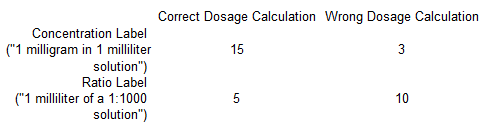The following data lists the number of correct and wrong dosage amounts calculated by 33 physicians. In a research​ experiment, a group of 18 physicians was given bottles of epinephrine labeled with a concentration of​ "1 milligram in 1 milliliter​ solution," and another group of 15 physicians was given bottles labeled with a ratio of​ "1 milliliter of a​ 1:1000 solution."

If one of the physicians is randomly​ selected, find the probability of getting one who made a correct dosage calculation or was given the bottle with a concentration label.

P(physician made a correct dosage calculation or was given the bottle with a concentration​ label) = 0.697.

P(correct dose calculation or with a concentration label)

= 20/33 + 18/33 – 15/33

= 0.696969697

18

What is wrong with the expression P(A) + P(Ā) = 0.5​?

Based on the rule of​ complements, the sum of​ P(A) and P(Ā) must always be​ 1, so that sum cannot be 0.5.

19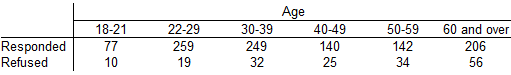The table below summarizes results from a study of people who refused to answer survey questions.

If one of the subjects is randomly​ selected, what is the probability that the selected person refused to​ answer?

Does the probability value suggest that refusals are a problem for​ pollsters?

The probability that a randomly selected person refused to answer is 0.141.

P(refused)

= (total refused) ÷ (total people)

= 176 / 1249

= 0.1409127302

​Yes, a high refusal rate results in a sample that is not necessarily representative of the population, b ecause those who refuse may well constitute a particular group with opinions different from others.

20

In a computer instant messaging​ survey, respondents were asked to choose the most fun way to​ flirt, and it found that ​P(D) = 0.620​, where D is directly in person.

If someone is randomly​ selected, what does P(D(–)) ​represent, and what is its​ value?

P(D(–)) is the probability of randomly selecting someone who does not choose a direct​ in-person encounter as the most fun way to flirt.

P(D(–)) = 0.380

1 – 0.620 = 0.38

21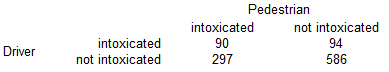The following data summarizes results from 1067 pedestrian deaths that were caused by accidents. If one of the pedestrian deaths is randomly​ selected, find the probability that the pedestrian was intoxicated or the driver was intoxicated.

​P(pedestrian was intoxicated or driver was ​intoxicated) = 0.451

= (90 + 297 + 94) ÷ 1067

= 0.4507966261

22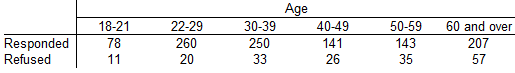The table below summarizes results from a study of people who refused to answer survey questions. A market researcher is not interested in refusals or subjects below 22 years of age or over 59.

Find the probability that the selected person refused to answer or is below 22 or is older than 59.

The probability that a randomly selected person refused to answer the survey question or is below 22 or is older than 59 is 0.370.

= [ (total refused) + (ages below 22 & over 59 who responded) ] ÷ (total people)

= [ (182) + (78 + 207) ] ÷ 1267

= 467 / 1261

= 0.3703409992

23

Pollsters are concerned about declining levels of cooperation among persons contacted in surveys. A pollster contacts 71 people in the​ 18-21 age bracket and finds that 66 of them respond and 5 refuse to respond. When 284 people in the​ 22-29 age bracket are​ contacted, 245 respond and 39 refuse to respond. Suppose that one of the 355 people is randomly selected.

Find the probability of getting someone in the 18 dash 21 age bracket or someone who refused to respond.

P(person is in the 18-21 age bracket or refused to respond​) = 0.310

= [(ages 18-21) + (other age brackets who refused)] ÷ (total people)

= (71 + 39) ÷ 355

= 0.3098591549

24

Determine whether the two events are disjoint for a single trial.​ (Hint: Consider​ "disjoint" to be equivalent to​ "separate" or​ "not overlapping".)

Randomly selecting a piano from the instrument assembly line and getting one that is free of defects.

Randomly selecting a piano from the instrument assembly line and getting one with a missing key.

The events are disjoint. They cannot occur at the same time.

25

Determine whether the two events are disjoint for a single trial.​ (Hint: Consider​ "disjoint" to be equivalent to​ "separate" or​ "not overlapping".)

Receiving a phone call from a volunteer survey subject who believes that there is solid evidence of global warming.

Receiving a phone call from a volunteer survey subject who is opposed to stem cell research.

The events are not disjoint. They can occur at the same time.

26

What does​ P(B|A) represent?

The probability of event B occurring after it is assumed that event A has already occurred

27

A tire company produced a batch of 6,900 tires that includes exactly 250 that are defective.

a. If 4 tires are randomly selected for installation on a​ car, what is the probability that they are all​ good?

b. If 100 tires are randomly selected for shipment to an​ outlet, what is the probability that they are all​ good?

c. Should this outlet plan to deal with defective tires returned by​ consumers?

a. 0.863

(6650/6900)4 = 0.8627604301

b. 0.025

(6650/6900)100 = 0.0249606251

c. Yes, because there is a very small chance that all 100 tires are good.

28

For the given pair of events A and​ B, complete parts​ (a) and​ (b) below.

​A: When a baby is​ born, it is a boy.

​B: When a 5​-sided die is​ rolled, the outcome is 4.

a. Determine whether events A and B are independent or dependent.​ (If two events are technically dependent but can be treated as if they are independent according to the​ 5% guideline, consider them to be​ independent.)

b. Find​ P(A and​ B), the probability that events A and B both occur.

a. The two events are independent because the occurrence of one does not affect the probability of the occurrence of the other.

b. The probability that events A and B both occur is 0.1000.

(1/2) x (1/5) = 0.1

29

For the given pair of events A and​ B, complete parts​ (a) and​ (b) below.

​A: A marble is randomly selected from a bag containing 11 marbles consisting of 1​ red, 4 ​blue, and 6 green marbles. The selected marble is one of the green marbles.​

B: A second marble is selected and it is the 1 red marble in the bag.

a. Determine whether events A and B are independent or dependent.​ (If two events are technically dependent but can be treated as if they are independent according to the​ 5% guideline, consider them to be​ independent.)

b. Find​ P(A and​ B), the probability that events A and B both occur.

a. The two events are dependent because the occurrence of one affects the probability of the occurrence of the other and the​ 5% guideline cannot be applied in this case.

b. The probability that events A and B both occur is 0.0545.

(6/11) x (1/10) = 0.0545454545

30

Describe what the notation P(B|A)represents.

The probability of event B​ occurring, given that event A has already occurred.

31

The principle of redundancy is used when system reliability is improved through redundant or backup components. Assume that a​ student's alarm clock has a 8.4​% daily failure rate.

a. What is the probability that the​ student's alarm clock will not work on the morning of an important final​ exam?

b. If the student has two such alarm​ clocks, what is the probability that they both fail on the morning of an important final​ exam?

c. What is the probability of not being awakened if the student uses three independent alarm​ clocks?

d. Do the second and third alarm clocks result in greatly improved​ reliability?

a. 0.084

b. 0.00726

0.0842 = 0.007056

c. 0.00059

0.0843 = 0.000592704

d. Yes, because total malfunction would not be​ impossible, but it would be unlikely.

32

Which word is associated with multiplication when computing​ probabilities?

And

33

Assume that a company hires employees on Mondays, Tuesdays, or Wednesdays with equal likelihood.

a. If two different employees are randomly​ selected, what is the probability that they were both hired on a Monday​?

b. If two different employees are randomly​ selected, what is the probability that they were both hired on the same day of the week​?

c. What is the probability that 7 people in the same department were all hired on the same day of the week​?

d. Is such an event​ unlikely?

a. The probability is 1/9 .

b. The probability is 1/3 .

c. The probability is 1/729 .

d. Yes, because the probability that all 7 people were hired on the same day of the week is less than or equal to 0.05.

34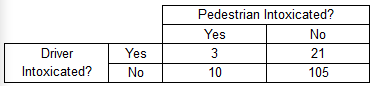The data in the following table summarize results from 139 pedestrian deaths that were caused by accidents.

If three different deaths are randomly selected without​ replacement, find the probability that they all involved intoxicated drivers.

Is such an event​ unlikely?

The probability is 0.004621.

(24/139) x (23/138) x (22/137) = 0.0046211206

Yes, because its probability is less than 0.05.

35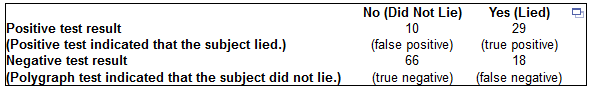The accompanying table contains the results from experiments with a polygraph instrument.

a. Four of the test subjects are randomly selected with​ replacement, and they all had true negative test results. Is such an event​ unlikely?

b. Four of the test subjects are randomly selected without​ replacement, and they all had true negative test results. Is such an event​ unlikely?

a. The probability that all four test subjects had a true negative test result when they are randomly selected with replacement is 0.083.

No, because the probability of the event is greater than 0.05.

(66/123)4 = 0.0829001462

b. The probability that all four test subjects had a true negative test result when they are randomly selected without replacement is 0.079.

No, because the probability of the event is greater than 0.05.

(66/123) x (65/122) x (64/121) x (63/120) = 0.0793864272